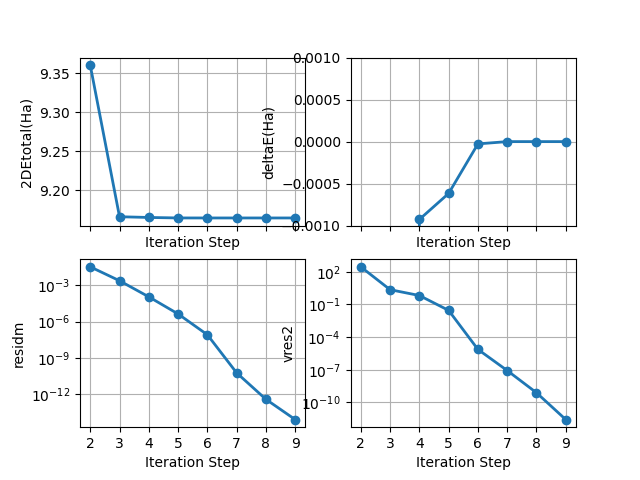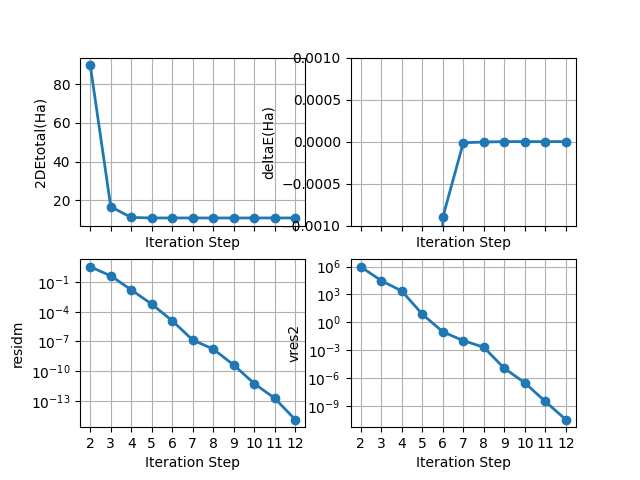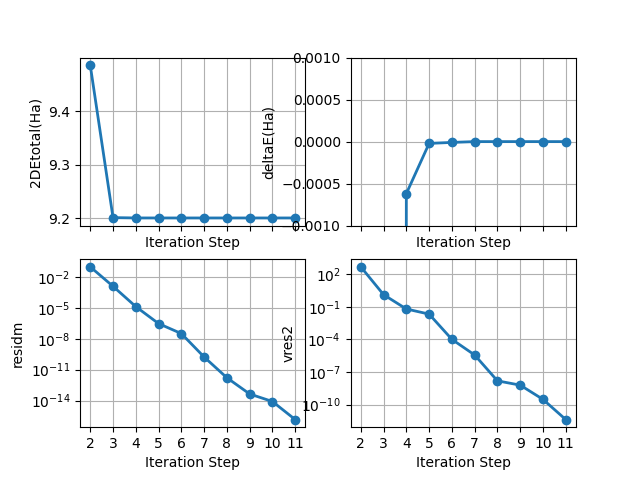# DFPT SCF cycle¶

This example shows how to plot the results of the DFPT self-consistent cycle reported in the main output file.

•••```from abipy.abilab import abiopen
import abipy.data as abidata

# Open the output file with DFPT calculations (Note the .abo extension).
# Alternatively, one can use `abiopen.py run.abo -nb` to generate a jupyter notebook.
abo = abiopen(abidata.ref_file("refs/gs_dfpt.abo"))

# Plot all SCF-GS sections found in the output file.
while True:
dfpt_cycle = abo.next_d2de_scf_cycle()
if dfpt_cycle is None: break
dfpt_cycle.plot()

# If timopt -1, we can extract the timing and plot the data.
#timer = abo.get_timer()
#timer.plot_pie()

abo.close()
```

Total running time of the script: ( 0 minutes 0.800 seconds)

Gallery generated by Sphinx-Gallery